### 前言

• 本文为深度学习500问项目的第五章，主要总结了CNN的一些必备知识点。

# 第五章 卷积神经网络（CNN）

## 5.1 卷积神经网络的组成层

CNN层次结构输出尺寸作用

$W_1\times H_1\times 3$对应原始图像或经过预处理的像素值矩阵，3对应RGB图像的通道;$K$表示卷积层中卷积核（滤波器）的个数;$W_2\times H_2$ 为池化后特征图的尺度，在全局池化中尺度对应$1\times 1$;$(W_2 \cdot H_2 \cdot K)$是将多维特征压缩到1维之后的大小，$C$对应的则是图像类别个数。

### 5.1.2 卷积层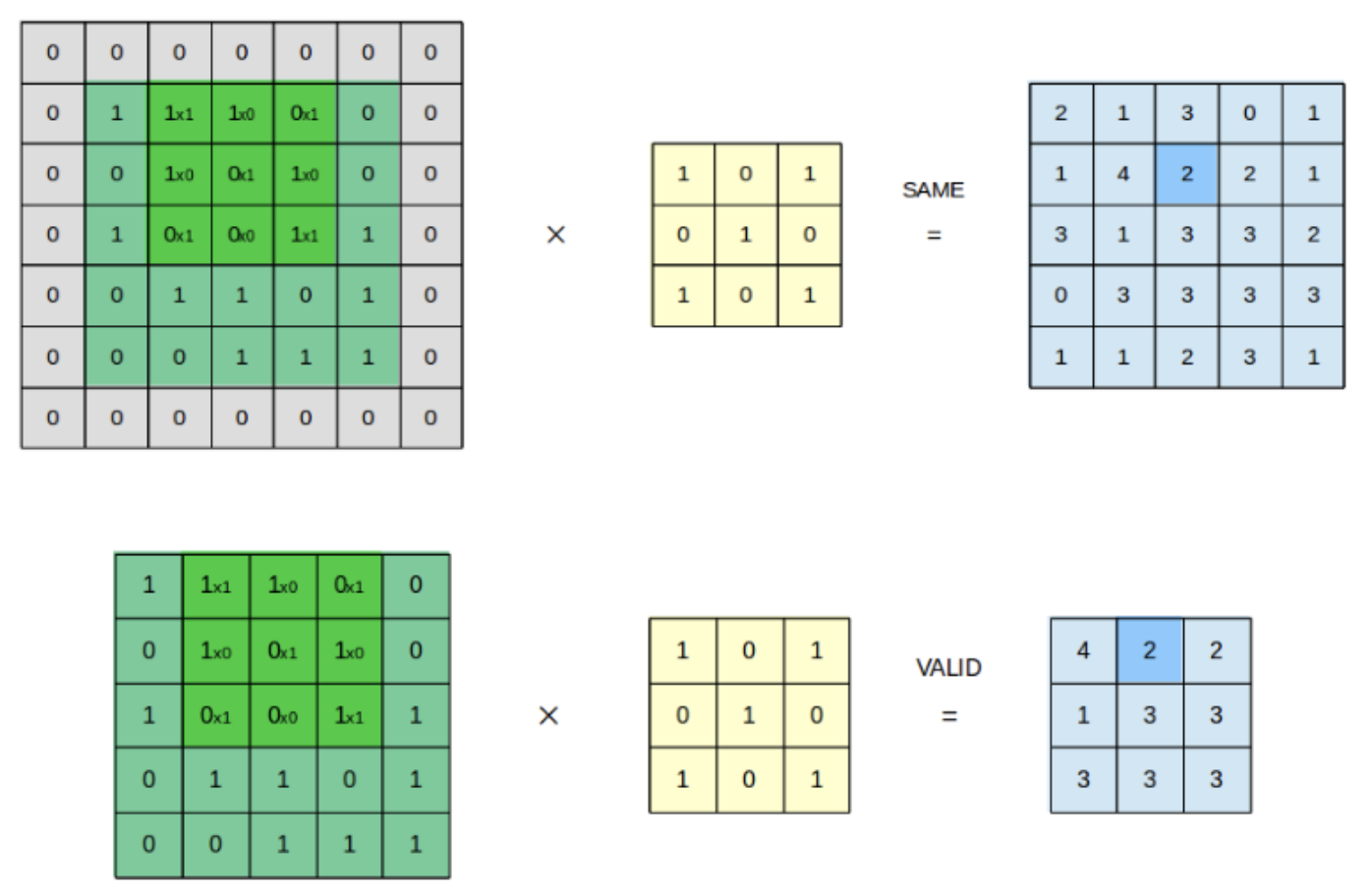## 5.3 卷积层有哪些基本参数？

$Od =\begin{cases} \lceil \frac{(I_d - k{size})+ 1)}{s}\rceil ,& \text{padding=VALID}\ \lceil \frac{I_d}{s}\rceil,&\text{padding=SAME} \end{cases}$

## 5.5 二维卷积与三维卷积有什么区别？

• 二维卷积
二维卷积操作如图5.3所示，为了更直观的说明，分别展示在单通道和多通道输入中，对单个通道输出的卷积操作。在单通道输入的情况下，若输入卷积核尺寸为 $(k_h, k_w, 1)$，卷积核在输入图像的空间维度上进行滑窗操作，每次滑窗和 $(k_h, k_w)$窗口内的值进行卷积操作，得到输出图像中的一个值。在多通道输入的情况下，假定输入图像特征通道数为3，卷积核尺寸则为$(k_h, k_w, 3)$，每次滑窗与3个通道上的$(k_h, k_w)$窗口内的所有值进行卷积操作，得到输出图像中的一个值。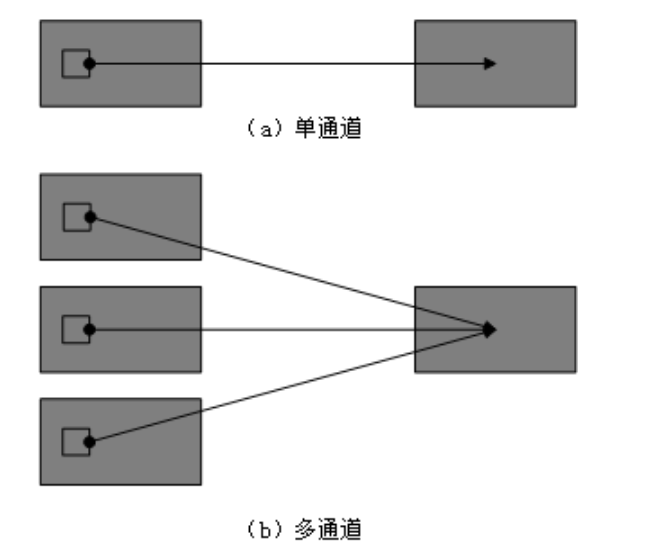• 三维卷积
3D卷积操作如图所示，同样分为单通道和多通道，且假定只使用1个卷积核，即输出图像仅有一个通道。对于单通道输入，与2D卷积不同之处在于，输入图像多了一个深度(depth)维度，卷积核也多了一个$k_d$维度，因此3D卷积核的尺寸为$(k_h, k_w, k_d)$，每次滑窗与$(k_h, k_w, k_d)$窗口内的值进行相关操作，得到输出3D图像中的一个值。对于多通道输入，则与2D卷积的操作一样，每次滑窗与3个channels上的$(k_h, k_w, k_d)$窗口内的所有值进行相关操作，得到输出3D图像中的一个值。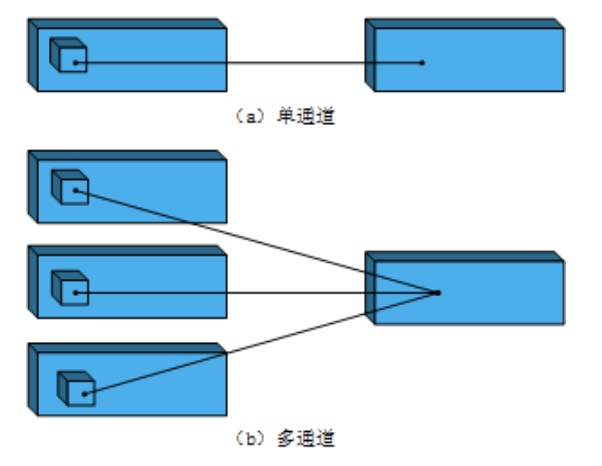## 5.7 有哪些池化方法？

SPPNet$^{}$就引入了空间池化的组合，对不同输出尺度采用不同的滑窗大小和步长以确保输出尺度相同$(win_{size}=\lceil \frac{in}{out}\rceil; stride=\lfloor \frac{in}{out}\rfloor; )$，同时用如金字塔式叠加的多种池化尺度组合，以提取更加丰富的图像特征。常用于多尺度训练和目标检测中的区域提议网络(Region Proposal Network)的兴趣区域(Region of Interest)提取

## 5.8 $1\times1$卷积作用

NIN(Network in Network)$^{}$是第一篇探索$1\times1$卷积核的论文，这篇论文通过在卷积层中使用MLP替代传统线性的卷积核，使单层卷积层内具有非线性映射的能力，也因其网络结构中嵌套MLP子网络而得名NIN。NIN对不同通道的特征整合到MLP自网络中，让不同通道的特征能够交互整合，使通道之间的信息得以流通，其中的MLP子网络恰恰可以用$1\times1$的卷积进行代替。

GoogLeNet$^{}$则采用$1\times1$卷积核来减少模型的参数量。在原始版本的Inception模块中，由于每一层网络采用了更多的卷积核，大大增加了模型的参数量。此时在每一个较大卷积核的卷积层前引入$1\times1$卷积，可以通过分离通道与宽高卷积来减少模型参数量。以图5.2为例，在不考虑参数偏置项的情况下，若输入和输出的通道数为$C_1=16$，则左半边网络模块所需的参数为$(1\times1+3\times3+5\times5+0)\times C_1\times C_1=8960$；假定右半边网络模块采用的$1\times1$卷积通道数为$C_2=8$$(满足C_1>C_2)$，则右半部分的网络结构所需参数量为$(1\times1\times (3C_1+C_2)+3\times3\times C_2 +5\times5\times C_2)\times C_1=5248$ ，可以在不改变模型表达能力的前提下大大减少所使用的参数量。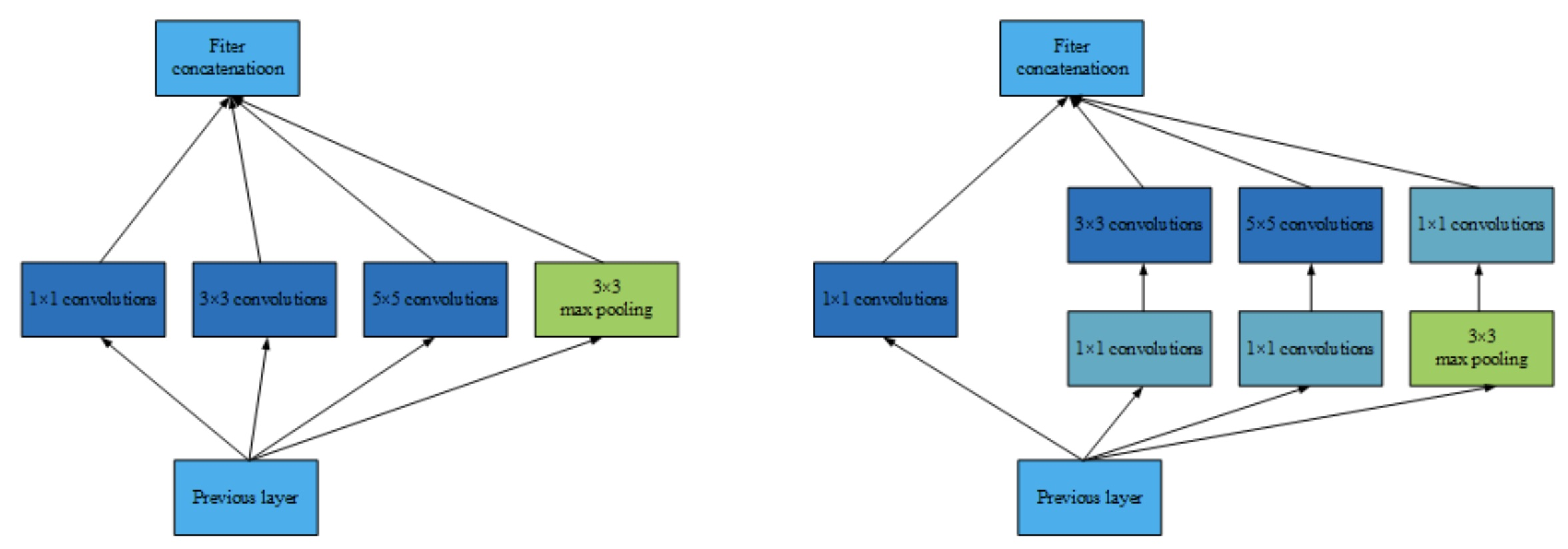• 实现信息的跨通道交互和整合。
• 对卷积核通道数进行降维和升维，减小参数量。

## 5.11 每层卷积是否只能用一种尺寸的卷积核？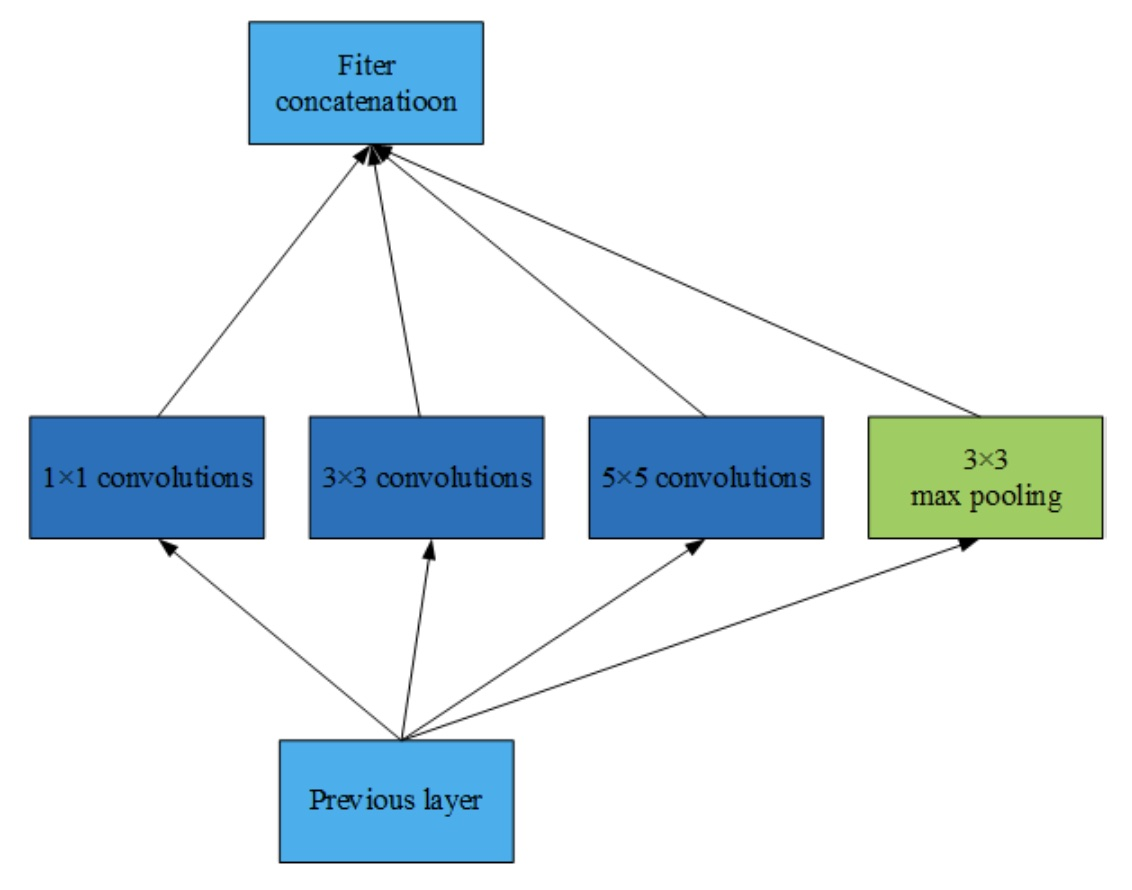## 5.12 怎样才能减少卷积层参数量？

• 使用堆叠小卷积核代替大卷积核：VGG网络中2个$3\times 3$的卷积核可以代替1个$5\times 5$的卷积核
• 使用分离卷积操作：将原本$K\times K\times C$的卷积操作分离为$K\times K\times 1$和$1\times1\times C$的两部分操作
• 添加$1\times 1$的卷积操作：与分离卷积类似，但是通道数可变，在$K\times K\times C_1$卷积前添加$1\times1\times C_2$的卷积核（满足$C_2 <C_1$）
• 在卷积层前使用池化操作：池化可以降低卷积层的输入特征维度

## 5.13 在进行卷积操作时，必须同时考虑通道和区域吗？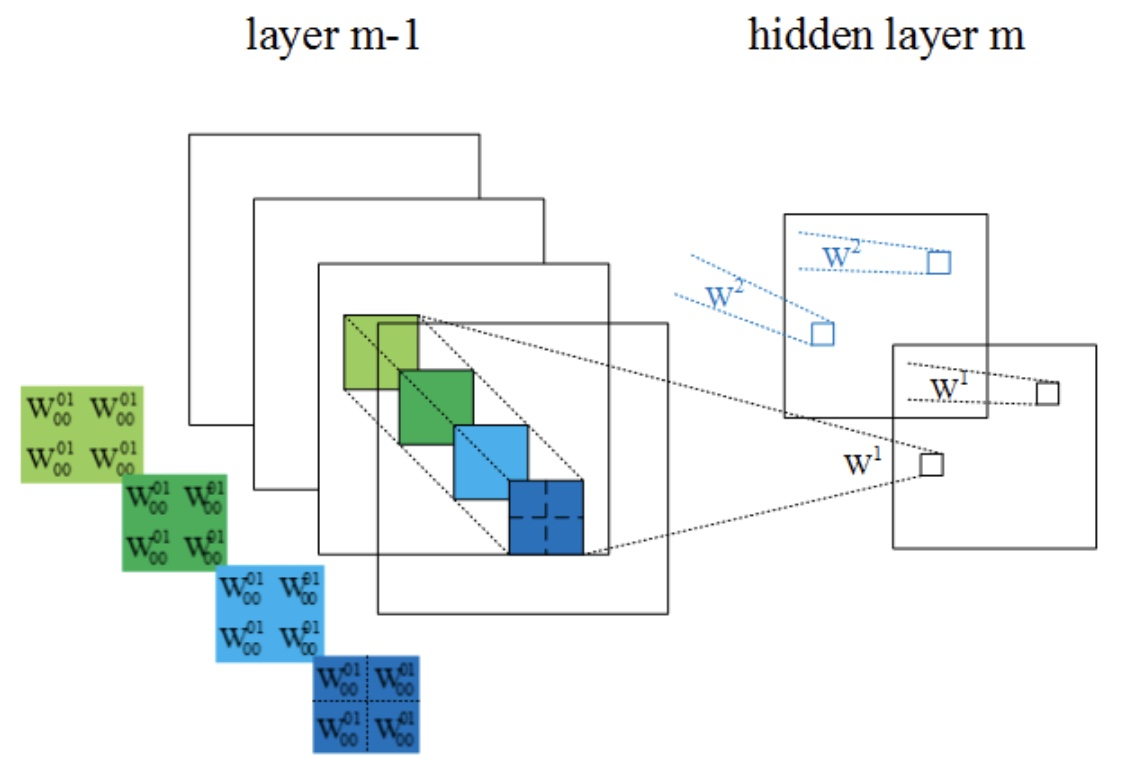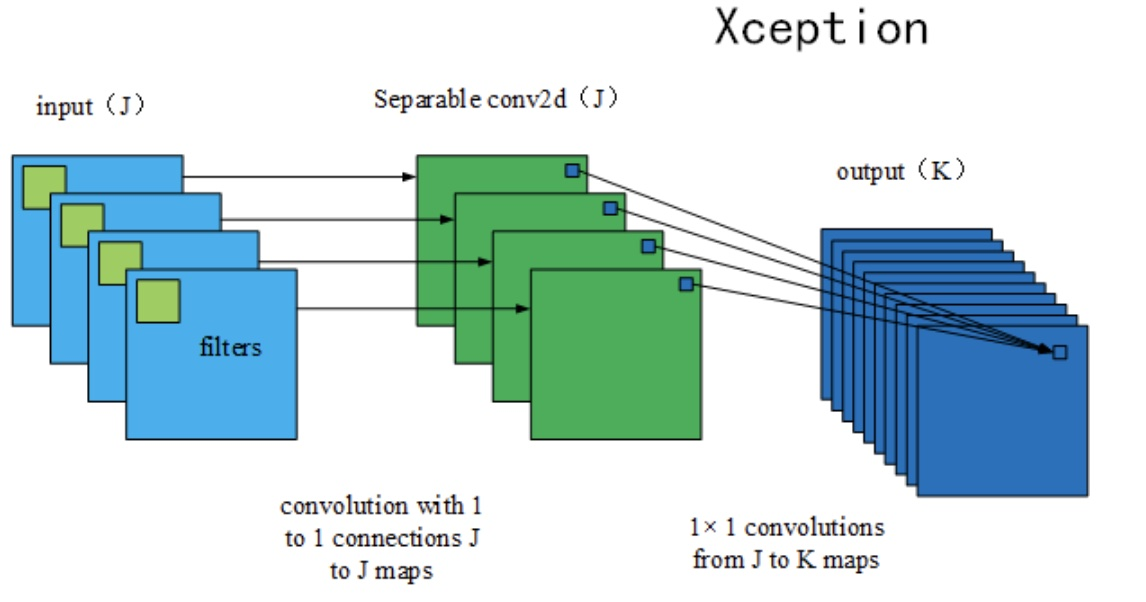## 5.14 采用宽卷积的好处有什么？

‘SAME’填充通常采用零填充的方式对卷积核不满足整除条件的输入特征进行补全，以使卷积层的输出维度保持与输入特征维度一致；’VALID’填充的方式则相反，实际并不进行任何填充，在输入特征边缘位置若不足以进行卷积操作，则对边缘信息进行舍弃，因此在步长为1的情况下该填充方式的卷积层输出特征维度可能会略小于输入特征的维度。此外，由于前一种方式通过补零来进行完整的卷积操作，可以有效地保留原始的输入特征信息。

## 5.15 理解转置卷积与棋盘效应

### 5.15.1 标准卷积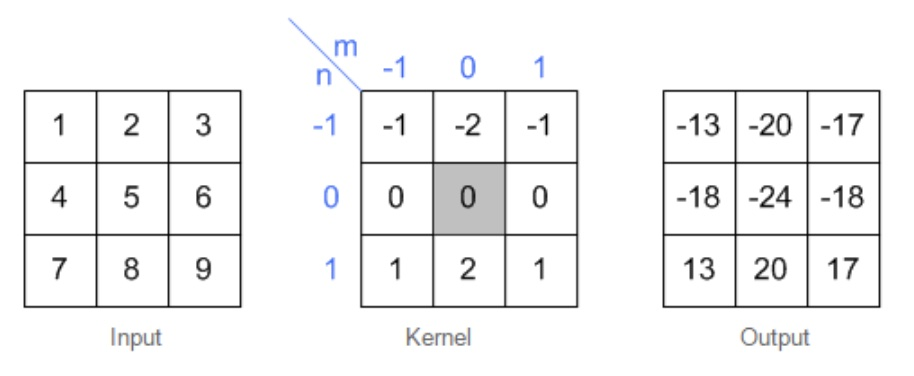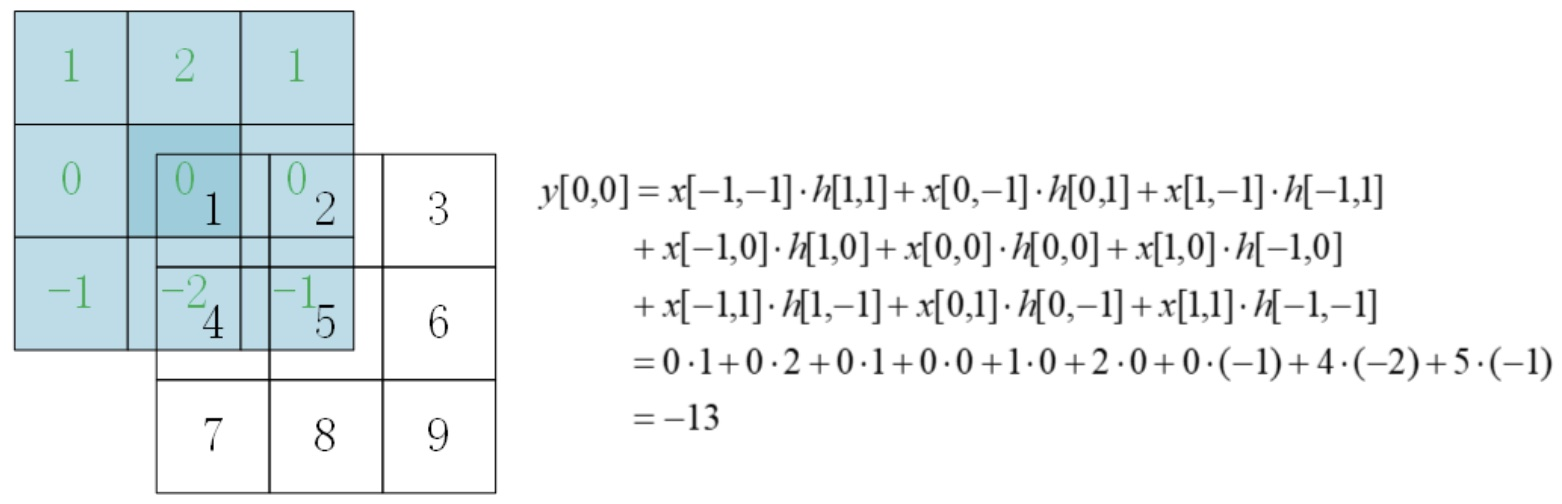### 5.15.2 转置卷积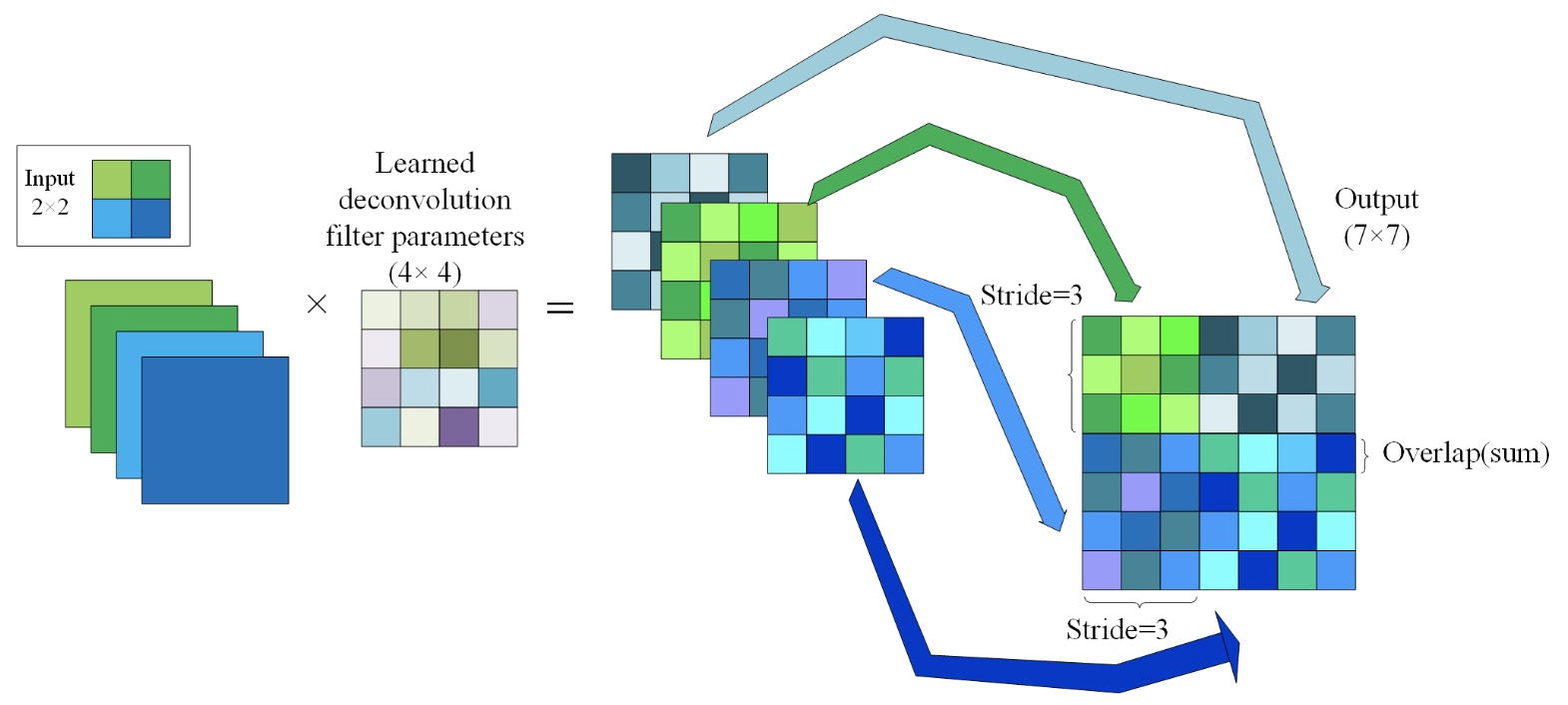1. 输入图片每个像素进行一次full卷积，根据full卷积大小计算可以知道每个像素的卷积后大小为 1+4-1=4， 即4x4大小的特征图，输入有4个像素所以4个4x4的特征图

2. 将4个特征图进行步长为3的相加； 输出的位置和输入的位置相同。步长为3是指每隔3个像素进行相加，重叠部分进行相加，即输出的第1行第4列是由红色特阵图的第一行第四列与绿色特征图的第一行第一列相加得到，其他如此类推。

可以看出翻卷积的大小是由卷积核大小与滑动步长决定， in是输入大小， k是卷积核大小， s是滑动步长， out是输出大小 得到 out = (in - 1) s + k 上图过程就是， (2 - 1) 3 + 4 = 7。

## 5.18 卷积神经网络在不同领域的应用

### 5.18.2 区别

1. 区域不变性：滤波器在每层的输入向量(图像)上滑动，检测的是局部信息，然后通过pooling取最大值或均值。pooling这步综合了局部特征，失去了每个特征的位置信息。这很适合基于图像的任务，比如要判断一幅图里有没有猫这种生物，你可能不会去关心这只猫出现在图像的哪个区域。但是在NLP里，词语在句子或是段落里出现的位置，顺序，都是很重要的信息。
2. 局部组合性：CNN中，每个滤波器都把较低层的局部特征组合生成较高层的更全局化的特征。这在CV里很好理解，像素组合成边缘，边缘生成形状，最后把各种形状组合起来得到复杂的物体表达。在语言里，当然也有类似的组合关系，但是远不如图像来的直接。而且在图像里，相邻像素必须是相关的，相邻的词语却未必相关。

## 5.19 卷积神经网络凸显共性的方法

### 5.19.1 局部连接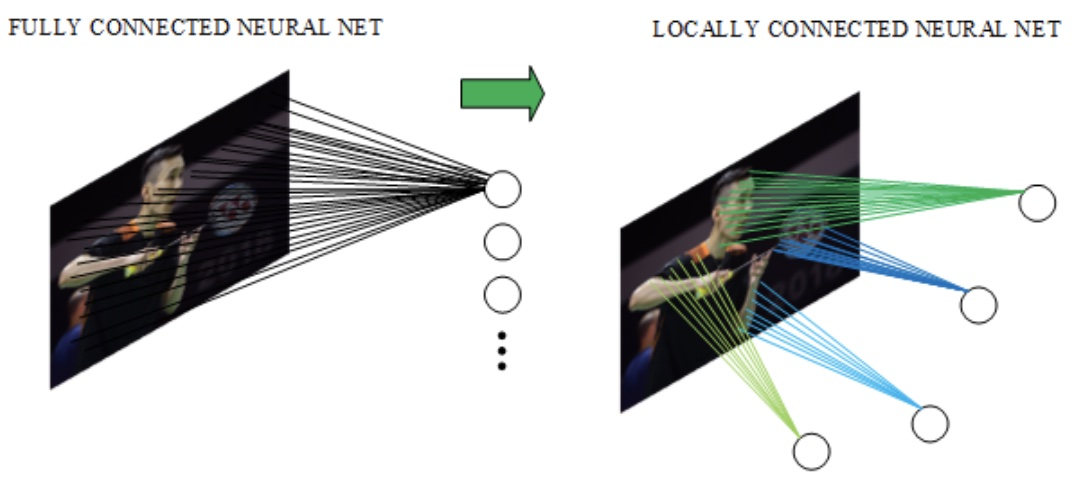### 5.19.2 权值共享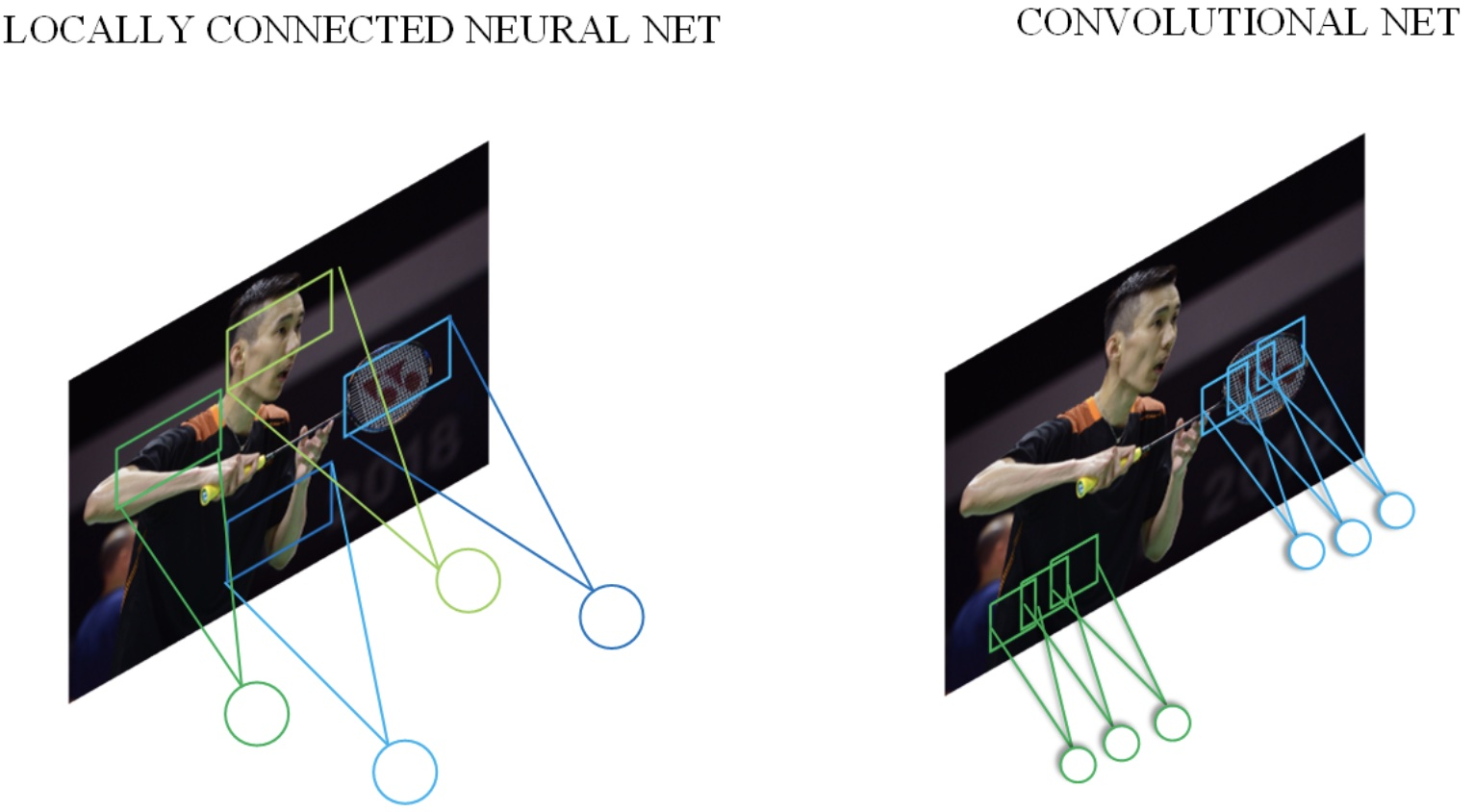### 5.19.3 池化操作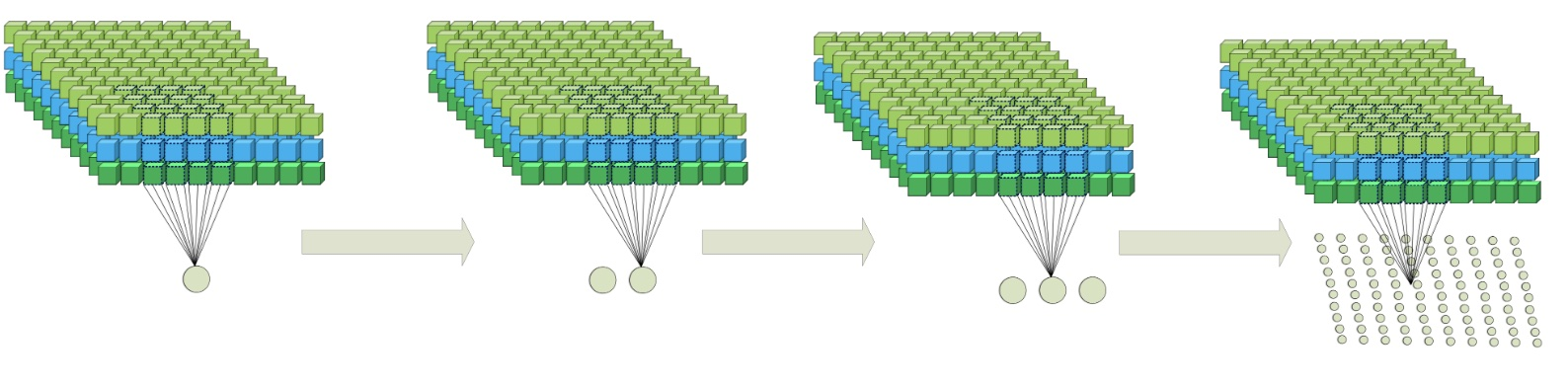## 5.20 全连接、局部连接、全卷积与局部卷积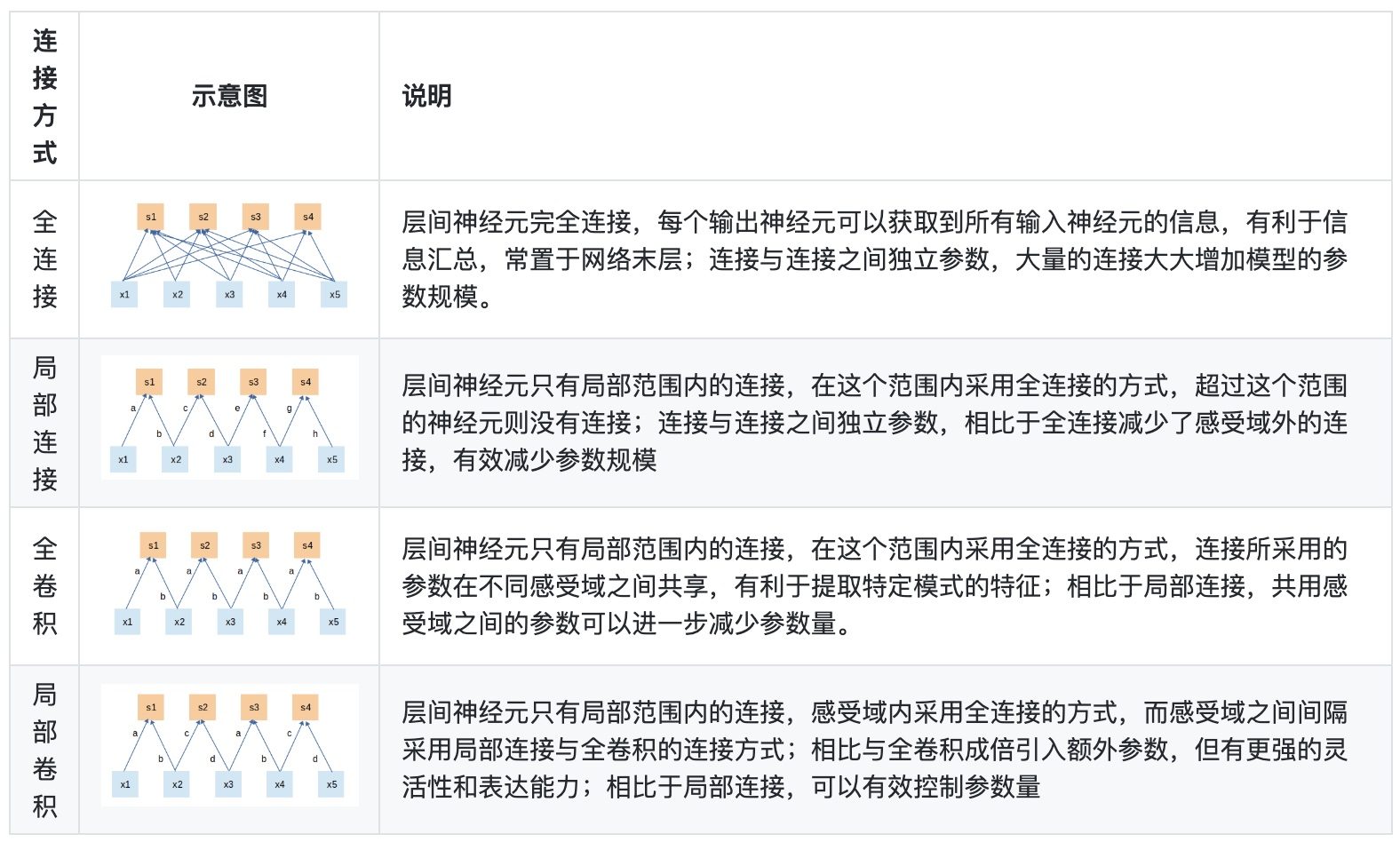## 5.21 局部卷积的应用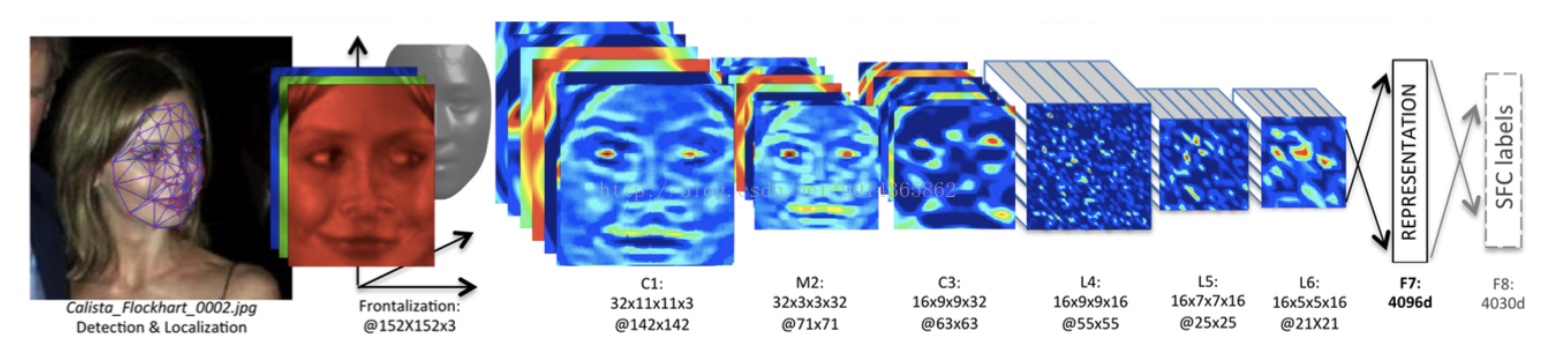Conv：32个11×11×3的卷积核，

Max-pooling: 3×3，stride=2，

Conv: 16个9×9的卷积核，

Local-Conv: 16个9×9的卷积核，

Local-Conv: 16个7×7的卷积核，

Local-Conv: 16个5×5的卷积核，

Fully-connected: 4096维，

Softmax: 4030维。

（1）对齐的人脸图片中，不同的区域会有不同的统计特征，因此并不存在特征的局部稳定性，所以使用相同的卷积核会导致信息的丢失。

（2）不共享的卷积核并不增加inference时特征的计算量，仅会增加训练时的计算量。

NetVLAD的一个输入是一个W x H x D的图像特征，例如VGG-Net最后的3 x 3 x 512这样的矩阵，在网络中还需加一个维度为Batchsize。

NetVLAD还需要另输入一个标量K即表示VLAD的聚类中心数量，它主要是来构成一个矩阵C，是通过原数据算出来的每一个$W \times H$特征的聚类中心，C的shape即$C: K \times D$，然后根据三个输入，VLAD是计算下式的V:## 参考文献

 卷积神经网络研究综述[J]. 计算机学报, 2017, 40(6):1229-1251.

 常亮, 邓小明, 周明全,等. 图像理解中的卷积神经网络[J]. 自动化学报, 2016, 42(9):1300-1312.

 Chua L O. CNN: A Paradigm for Complexity[M]// CNN a paradigm for complexity /. 1998.

 He K, Gkioxari G, Dollar P, et al. Mask R-CNN[J]. IEEE Transactions on Pattern Analysis & Machine Intelligence, 2017, PP(99):1-1.

 Hoochang S, Roth H R, Gao M, et al. Deep Convolutional Neural Networks for Computer-Aided Detection: CNN Architectures, Dataset Characteristics and Transfer Learning[J]. IEEE Transactions on Medical Imaging, 2016, 35(5):1285-1298.

 许可. 卷积神经网络在图像识别上的应用的研究[D]. 浙江大学, 2012.

 陈先昌. 基于卷积神经网络的深度学习算法与应用研究[D]. 浙江工商大学, 2014.

 cs231n 动态卷积图：http://cs231n.github.io/assets/conv-demo/index.html

 Krizhevsky A, Sutskever I, Hinton G E. Imagenet classification with deep convolutional neural networks[C]//Advances in neural information processing systems. 2012: 1097-1105.

 Sun Y, Wang X, Tang X. Deep learning face representation from predicting 10,000 classes[C]//Computer Vision and Pattern Recognition (CVPR), 2014 IEEE Conference on. IEEE, 2014: 1891-1898.

 魏秀参.解析深度学习——卷积神经网络原理与视觉实践[M].电子工业出版社, 2018

 Jianxin W U , Gao B B , Wei X S , et al. Resource-constrained deep learning: challenges and practices[J]. Scientia Sinica(Informationis), 2018.

 Arandjelovic R , Gronat P , Torii A , et al. [IEEE 2016 IEEE Conference on Computer Vision and Pattern Recognition (CVPR) - Las Vegas, NV, USA (2016.6.27-2016.6.30)] 2016 IEEE Conference on Computer Vision and Pattern Recognition (CVPR) - NetVLAD: CNN Architecture for Weakly Supervised Place Recognition[C]// 2016:5297-5307.

-------------The End-------------